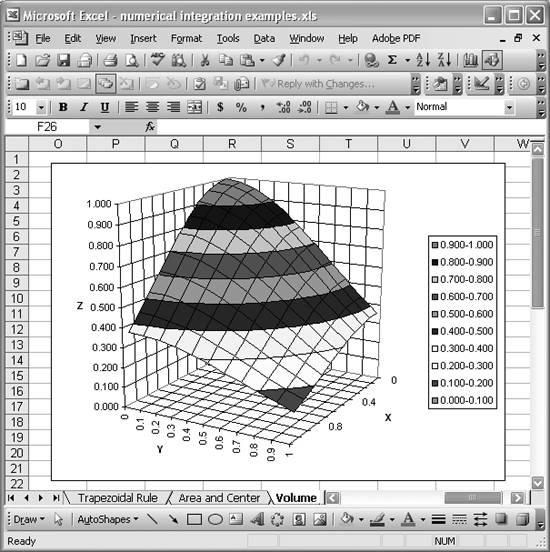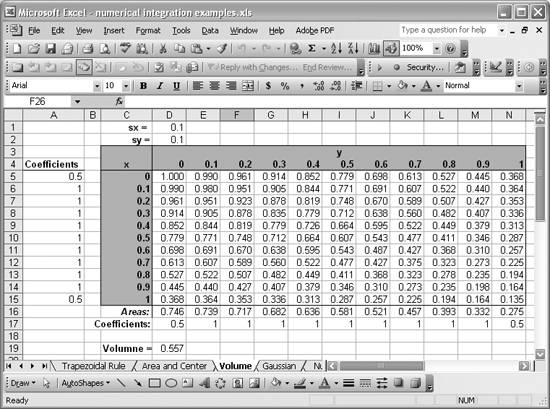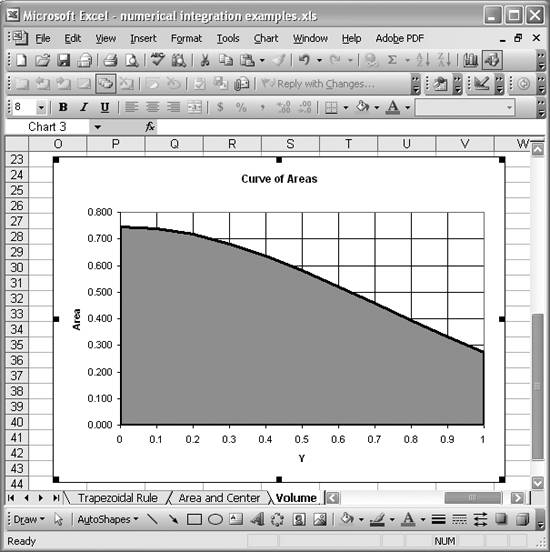# Dealing with Double Integrals

Problem

You need to numerically integrate a double integral (for example, to compute the volume of some arbitrary shape or the volume under a surface).

Solution

Separate the problem into two parts, each involving only single integrals, and then apply the numerical integration techniques discussed earlier in this chapter.

Discussion

Computing multiple integrals numerically can be challenging, especially when triple or higher integrals and involved. To the best of my knowledge, there are no general-purpose numerical integration formulas that handle multiple integrals. There is a method called Monte Carlo integration that involves taking a lot of random samples within the volume or domain being integrated and using the distribution of those samples to estimate the volume or whatever is of interest in higher dimensions. Alternatively, you can break up a multiple integral into successive single integrals and apply the standard numerical integration techniques discussed in this chapter.

To demonstrate this latter approach, consider the surface shown in Figure 10-3.

Figure 10-3. 3D surfaceLet's assume you want to compute the volume under that surface bounded by y = [0,1] and x = [0,1]. This is a three-dimensional problem, but instead of computing a multiple integral, you can compute a series of single integrals.

The first step is to consider cross-sections of this surface at successive y-values. Each cross-section, at each y-value, will yield a curve. We can compute the area under each of these curves using a standard integration rule. You'll end up with a new set of data that represents cross-sectional areas as a function of y. You can then integrate the resulting curve of areas to get the volume under the surface.

Figure 10-4 shows the data that represents the surface in Figure 10-3.

The table contains z-values for corresponding x- and y-values. As outlined a moment ago, the first task is to compute cross-sectional areas corresponding to cross-sections

Figure 10-4. Data for 3D surfacetaken of the surface at each y-value. This means that for each y-value, held constant, we can integrate along the x-axis to compute the area under the cross-section curve.

The row labeled Areas on row 16 of the spreadsheet shows the results of integrating the cross-section curves for each y-value. I applied the trapezoidal rule here, using formulas like =\$D\$1*SUMPRODUCT(\$A\$5:\$A\$15,D5:D15). Cells D16 through N16 contain formulas just like this one to compute each cross-sectional area, that is, at each y-value.

If you plot the resulting cross-sectional areas versus the y-values, you end up with a curve like that shown in Figure 10-5.

This plot was generated using the y-data in the cell range D4:N4 and the area data in the cell range D16:N16. The area under this curve is the volume under the surface. So now all you need to do is integrate this cross-sectional area curve to get the volume. Cell D19 contains the formula =D2*SUMPRODUCT(D17:N17,D16:N16), which performs the integration of the cross-sectional area curve, yielding the volume under the surface.

That's all there is to it. This technique is fairly straightforward and readily implemented in Excel.

Figure 10-5. Cross-sectional area curveExcel Scientific and Engineering Cookbook (Cookbooks (OReilly))
ISBN: 0596008791
EAN: 2147483647
Year: N/A
Pages: 206
Authors: David M Bourg